# XOR Gate & XNOR Gates: Truth Table, Symbol & Boolean Expression

Contents## What is an XOR Gate?

An XOR gate (also known as an EOR, or EXOR gate) – pronounced as Exclusive OR gate – is a digital logic gate that gives a true (i.e. a HIGH or 1) output when the number of true inputs is odd. An XOR gate implements an exclusive OR, i.e., a true output result occurs if one – and only one – of the gate’s inputs is true. If both inputs are false (i.e. LOW or 0) or both inputs are true, the output is false.

XOR represents the inequality function, i.e. the output is true if the inputs are not alike; otherwise, the output is false. A common way to remember the XOR is “must have one or the other, but not both”.

Another way to look at an XOR gate: a modulo sum of two variables in a binary system looks like this: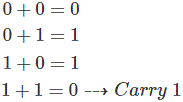The logic gate performs this modulo sum operation without including carry is known as XOR gate. An XOR gate is normally two inputs logic gate where the output is only logical 1 when only one input is logical 1. When both inputs are equal, either are 1 or both are 0, the output will be logical 0.

This is the reason an XOR gate is also called an anti-coincidence gate or inequality detector. This gate is called XOR or exclusive OR gate because its output is only 1 when its input is exclusively 1.

In digital electronics, other logic gates include NOT gates, OR gates, NAND gates, and NOR Gates.

## XOR Gate Truth Table

Truth tables list the output of a particular digital logic circuit for all the possible combinations of its inputs. The truth table of an XOR gate is given below: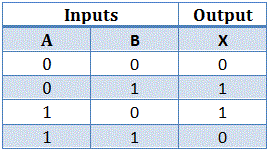The above truth table’s binary operation is known as exclusive OR operation. It is represented as A ⊕ B. The symbol of exclusive OR operation is represented by a plus ring surrounded by a circle ⊕.

## XOR Gate Circuit Diagram

The above expression, A ⊕ B can be simplified as,Let us prove the above expression.
In first case consider, A = 0 and B = 0.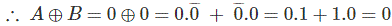In second case consider, A = 0 and B = 1.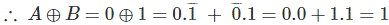In third case consider, A = 1 and B = 0.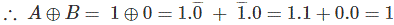In fourth case consider, A = 1 and B = 1.So it is proved that the Boolean expression for A ⊕ B is AB ̅ + ĀB, as this Boolean expression satisfied all output states with respect to an XOR gate’s inputs conditions.

From this Boolean expression, one can easily realize the logical circuit of an XOR gate, and this will be as shown,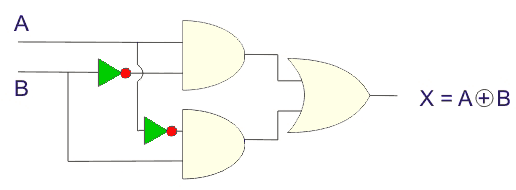## Logical Symbol of XOR Gate

An XOR gate is logically represented as,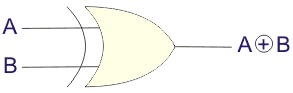## Multiple Input XOR Gate

Although XOR gates can only have two inputs, you can perform the XOR operation using any number of inputs (e.g. 3 input XOR operation or 4 input XOR operation).

More than two inputs XOR operation is that, when the odd number of inputs in the gate area 1, the output is 1, and when none or even the number of inputs is 1, the output is logical 0.

## 3 Input XOR Gate

Let us realize an XOR gate with three inputs A, B, and C.

Now, as per the definition of XOR operation with more than three inputs, the truth table would be,This truth table can be elaborated as,From the above-elaborated truth table, it is found that the XOR operation of three binary variables is equivalent to, XOR operation of one variable with the result of XOR operations of the other two variables.

From the above truth table,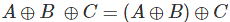## What is an XNOR Gate?

The XNOR gate (also known as a XORN’T, ENOR, EXNOR or NXOR) – and pronounced as Exclusive NOR – is a digital logic gate whose function is the logical complement of the exclusive OR gate (XOR gate). Logically, an XNOR gate is a NOT gate followed by an XOR gate.

The XOR operation of inputs A and B is A ⊕ B; therefore, XNOR operation those inputs will be (A + B) ̅. That means the output of the XOR gate is inverted in the XNOR gate.

In the XOR gate operation, the output is only 1 when only one input is 1. The output is logical 0 when both inputs are the same, meaning they are either 1 or 0. But in the XNOR gate, the inverse is true. Hence the output is 0 when only one input is 0, and the output is 1 when both inputs are the same (i.e. either two 0’s or two 1’s).

See the XNOR gate truth table below for a visual representation of this.

## XNOR Gate Truth Table

The truth table of the XNOR gate is shown below:The logical XNOR operation is represented by ⊙. That is a dot surrounded by a circle. The expression of XNOR operation between variables A and B is represented as A ⊙ B.
Now again, the truth table is satisfied by the equation AB + ĀB ̅.Hence, it is proved that A ⊙ B = AB + ĀB ̅. The same can be proved by using K-map also.

## XNOR Gate Circuit Diagram

The expression of XNOR operation can be realized by using two NOT gates, two AND gates, and one OR gate as followers,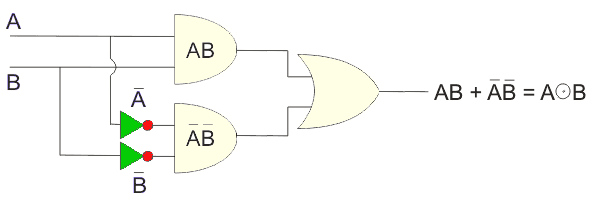The symbol of the XNOR gate: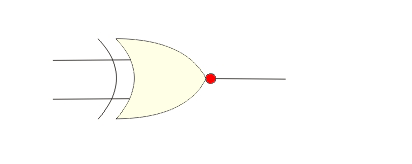## 3 Input XNOR Gate

Like the XOR gate, an XNOR gate only exists with two inputs, but for XNOR operation with more than two inputs, we have to use more than one XNOR gates.

XNOR operation with more than two inputs is like that. When there are odd numbers of inputs in high or logical 1 condition, the output will be 0; otherwise, the output will be 1.Now,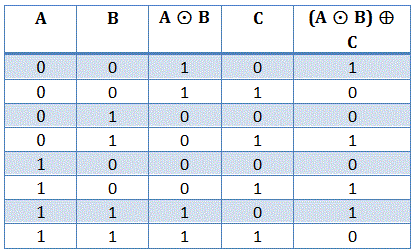From this elaborate truth table, the logical symbol of three inputs XNOR gate can be represented as,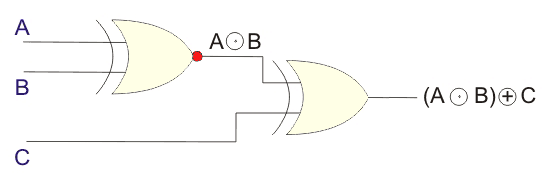## Applications of XOR Gates

The main application of the Exclusive OR gate is in the operation of half and full adder. If we look at the truth table carefully, we will find that the first three results are totally satisfying the process of binary addition. Still, in the last input sequence i.e. when both the inputs are 1, the result according to the rule of addition should be 0 with a carry 1. In the truth table, we are getting the desired 0 but a missing 1.

To solve this problem while designing an adder’s circuit, an AND gate is added to the Ex-OR gate in parallel. We will show the circuit of the adder in detail.From the above diagram, we can see that the two inputs are going through an Exclusive-OR gate and an AND gate parallelly in the half adder circuit. And with this circuit’s operation, we get the total process of binary addition smoothly.

Pseudo-random number generation – to model a linear feedback shift register, Ex-OR gates are used and generate a random sequence of bits.

Want To Learn Faster? 🎓
Get electrical articles delivered to your inbox every week.
No credit card required—it’s 100% free.